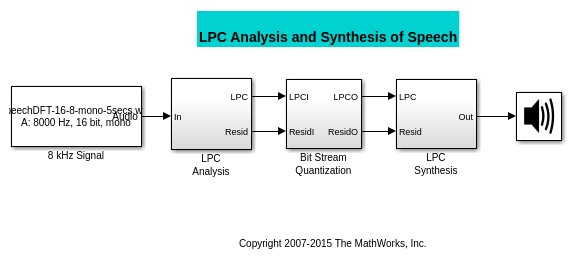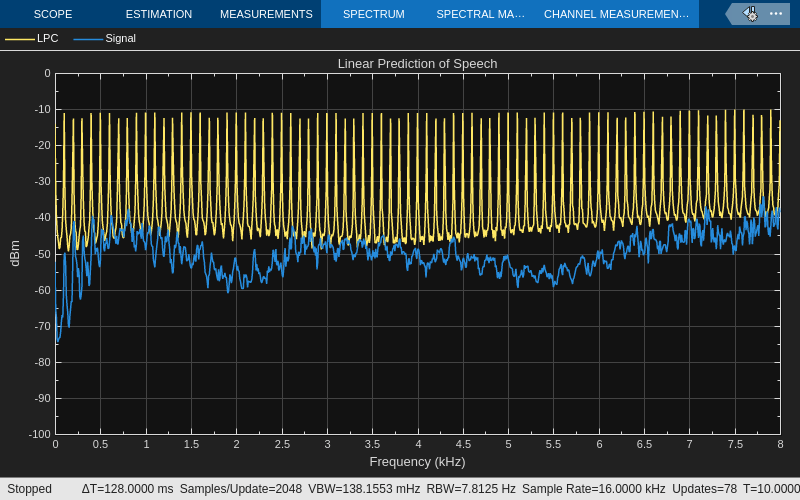# LPC Analysis and Synthesis of Speech

This example shows how to use the Levinson-Durbin and Time-Varying Lattice Filter blocks for low-bandwidth transmission of speech using linear predictive coding.

### Example Model### Example Description

The example consists of two parts: analysis and synthesis. The analysis portion 'LPC Analysis' is found in the transmitter section of the system. Reflection coefficients and the residual signal are extracted from the original speech signal and then transmitted over a channel. The synthesis portion 'LPC Synthesis', which is found in the receiver section of the system, reconstructs the original signal using the reflection coefficients and the residual signal.

In this simulation, the speech signal is divided into 20 ms frames (160 samples), with an overlap of 10 ms (80 samples). Each frame is windowed using a Hamming window. Eleventh-order autocorrelation coefficients are found, and then the reflection coefficients are calculated from the autocorrelation coefficients using the Levinson-Durbin algorithm. The original speech signal is passed through an analysis filter, which is an all-zero filter with coefficients same as the reflection coefficients obtained above. The output of the filter is the residual signal. This residual signal is passed through a synthesis filter which is the inverse of the analysis filter. The output of the synthesis filter is the original signal. This is played through the 'Audio Device Writer' block.

## SupportGet trial now